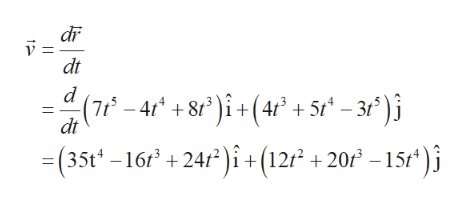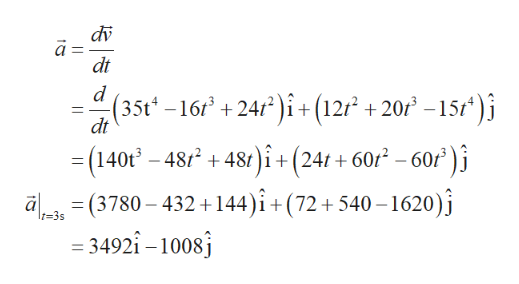# The 2-D Cartesian position vector r for a particle in motion is shown below in meters where t is in seconds:r =  7 t5 - 4 t4 + 8 t3  i   +   4 t3 + 5 t4 - 3 t5  jAt time = 3 seconds, determine the magnitude of the acceleration vector in m/s/s.

Question
26 views

The 2-D Cartesian position vector r for a particle in motion is shown below in meters where t is in seconds:

r =  7 t- 4 t+ 8 t3  i   +   4 t+ 5 t- 3 t5  j

At time = 3 seconds, determine the magnitude of the acceleration vector in m/s/s.

check_circle

Step 1

The velocity of particle ishelp_outlineImage Transcriptionclosedi dt d (7-4 +8 )i+ (4r +5 -3)i =(35t-16t +24/)i+(12 +20 -15t* )j dt fullscreen
Step 2

The acceleration of...help_outlineImage Transcriptionclosedv dt (35t -16 +24/i+ (12r +20f -15f* )j dt i+ (24t+ 602 - 60r)i (140t -48248t a(3780-432+144)i+(72+540-1620) = 3492î -1008 t-3s fullscreen

### Want to see the full answer?

See Solution

#### Want to see this answer and more?

Solutions are written by subject experts who are available 24/7. Questions are typically answered within 1 hour.*

See Solution
*Response times may vary by subject and question.
Tagged in

### Other